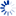MSTE
Office for Mathematics, Science, and Technology Education
College of Education @ University of Illinois

# Prime Number Determiner

This page presents the user with the chance to determine if a certain number is prime. The calculation is accurate for numbers 16 digits or shorter.

Please enter a number and then press the Determine button:

Enter number here:

 Check ALL Factors: Check This Many Factors: Reset the Form:

Result is:Elapsed Time:

Algorithm Output:

As you read the result, remember the typical divisibility tests for numbers to diagnose if they are prime or not.

A natural number is divisible by:

 2 if the number is even 3 if the sum of the digits is divisible by 3 4 if the last two digits are a number divisible by 4 5 if the last digit is 5 or 0 6 if the number is even and divisible by 3 8 if the number is divisible by 4 and the result is even 9 if the sum of the digits is divisible by 9 10 if the last digit is 0